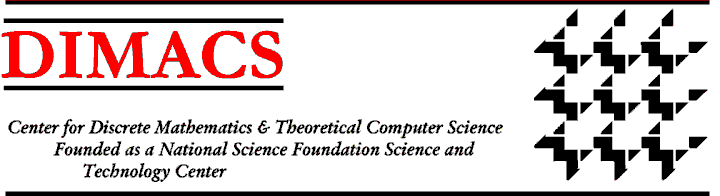The DIMACS/LAMSADE collaborative project includes the exchange of graduate students for extended visits. In November and December, 2004, two graduate students from LAMSADE, Bruno Escoffier and Meltem Ozturk, were in residence as DIMACS visitors. They interacted with Rutgers graduate students and faculty, and gave seminars on their research. DIMACS has selected two graduate students, Tiberius Bonates and Marcin Kaminski, to visit LAMSADE in Spring 2005.

Meltem Ozturk is working in decision analysis, constructing models to represent the problem situation (set of alternatives, criteria, attributes etc.) and the vision of the decision maker (his or her preferences, definition of rationality, etc.). She attempts to account for the dubious, uncertain, imprecise, inconsistent information and the complex nature of a decision maker's preferences (like hesitation between preference and indifference). In her models, uncertainties of information are represented by intervals and some extended preference structures to deal with the complex nature of preferences. Such orders introduce a third relation, "weak preference," which permits the decision maker to express hesitation between preference and indifference. She has used non-classical logics (especially a four valued one and its continuous extension) and fuzzy set theory to characterize such structures.

Bruno Escoffier is working on a method to evaluate how well a polynomial algorithm can approximate the solution to an NP-hard problem. Many well known combinatorial optimization problems are NP-hard, hence we cannot compute an optimal solution in polynomial time unless P=NP. In the polynomial approximation framework, one wants to devise algorithms that compute in polynomial time a feasible solution that is as good as possible. The most classical way to measure the quality of the solution A(I) on instance I is to compute the ratio between the value of A(I) and the optimal value opt(I). Bruno is studying a different approximation measure, called differential ratio, where the quality of A(I) is measured by looking at its relative position in the interval of feasible values [w(I),opt(I)]. Here, w(I) denotes the value of the worst solution. He has obtained approximation results for particular problems (such as TSP and SAT), as well as structural results, in order to determine the differences/similarities between the landscapes of standard and differential approximation.

Tiberius Bonates has been working under the supervision of Peter L. Hammer on optimization and combinatorial methods applied to machine learning. He has worked mainly on Boolean problems arising in the context of the classification method called Logical Analysis of Data. His main activities in this research have been to conceive and implement algorithms to solve such problems. Hammer and Bonates have been addressing some of these problems by the use of integer linear programming and heuristics. In some cases they are also interested in identifying combinatorial properties in datasets.

Marcin Kaminski's research interests lie in combinatorics, graph theory and their algorithmic applications. Having completed most of the required coursework in the last two years, since summer 2004 he has been working on exact graph coloring algorithms and related problems (maximal independent sets, maximal bipartite subgraphs). His other interests are in poset theory, probabilistic algorithms, and combinatorial optimization. At LAMSADE, the research of the Algorithmes et modles d'optimisation group is especially interesting to him, in particular polynomial time approximation coloring algorithms and probabilistic algorithms.

Photos from the luncheon:
Picture 1
Picture 2Previous: LAMSADE/DIMACS PartnershipDIMACS Homepage